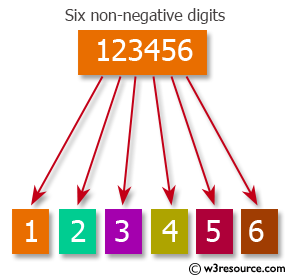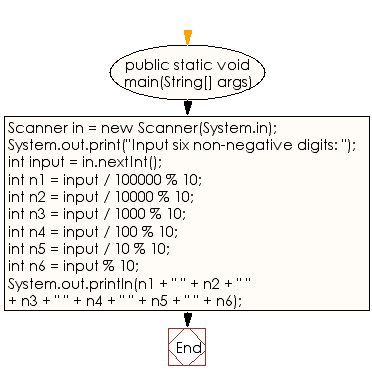﻿ Java exercises: Breaks an integer into a sequence of individual digits - w3resource# Java Data Type Exercises: Breaks an integer into a sequence of individual digits

## Java Data Type: Exercise-10 with Solution

Write a Java program to break an integer into a sequence of individual digits.

An integer is a number that can be written without a fractional component. For example, 12, 8, 0, and -2365 are integers, while 4.25, 57 1 / 2, and √3 are not

Test Data
Input six non-negative digits: 123456Sample Solution:

Java Code:

``````import java.util.Scanner;
public class Exercise11 {

public static void main(String[] args)
{
Scanner in = new Scanner(System.in);
System.out.print("Input six non-negative digits: ");
int input = in.nextInt();
int n1 = input / 100000 % 10;
int n2 = input / 10000 % 10;
int n3 = input / 1000 % 10;
int n4 = input / 100 % 10;
int n5 = input / 10 % 10;
int n6 = input % 10;
System.out.println(n1 + " " + n2 + " " + n3 + " " + n4 + " " + n5 + " " + n6);

}
}
```
```

Sample Output:

```Input six non-negative digits: 123456
1 2 3 4 5 6
```

Flowchart:Java Code Editor:

Improve this sample solution and post your code through Disqus

What is the difficulty level of this exercise?

﻿

## Java: Tips of the Day

Checks if a string is upper case:

```public static boolean isUpperCase(String input) {
return Objects.equals(input, input.toUpperCase());
}
```

Ref: https://bit.ly/39Hpo84